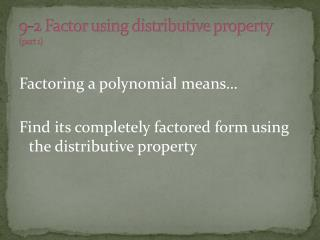DownloadDownload Presentation9-2 Factor using distributive property (part 1)

# 9-2 Factor using distributive property (part 1)

Download Presentation## 9-2 Factor using distributive property (part 1)

- - - - - - - - - - - - - - - - - - - - - - - - - - - E N D - - - - - - - - - - - - - - - - - - - - - - - - - - -
##### Presentation Transcript

1. 9-2 Factor using distributive property(part 1) Factoring a polynomial means… Find its completely factored form using the distributive property

2. What are the steps? • Find the GCF • Divide out the GCF • Put GCF on outside of ( ) • Leave everything else on the inside of ( )

3. Let's look at 12a2 + 16a

4. Let's look at 12a2 + 16a 12a2 + 16a / \ / \ 2 6 2 8 / \ / \ 2 3 2 4 /\ 2 2

5. 1. Find the GCF 12a2 + 16a / \ / \ 2 6 2 8 12a2 = 2*2*3*a*a / \ / \ 16a = 2*2*2*2*a 2 3 2 4 /\ GCF = 4a 2 2

6. 2. Divide out GCF 12a2 + 16a 4a 4a leaves 3a + 4

7. 3. Put GCF on outside of ( )4. Leave everything else inside the ( ) So, 12a2 + 16a = 4a ( 3a + 4 )

8. How about 18cd2 + 12c2d + 9cd First find the GCF Then divide it out Write the factors (GCF on outside and rest on inside)

9. How about 18cd2 + 12c2d + 9cd 18cd2 + 12c2d + 9cd / \ / \ / \ 2 9 2 6 3 3 / \ / \ 3 3 2 3 18cd2 = 2*3*3*c* d*d GCF 12c2d = 2*3*3*c*c*d 3cd 9cd = 3*3*c* d

10. So, 18cd2 + 12c2d + 9cd 3cd 3cd3cd 3cd ( 6d + 4c + 3 ) GCF ( what’s left)

11. How do we solve equations that contain polynomials? 1.Move all terms to the left of the (=) so that the polynomial is equal to zero. 2. Factor the left side of the polynomial. 3. Use the multiplication property of zero to solve. (the multiplication property of zero says: if two factors multiply to equal zero, then one or both factors must be equal to zero)

12. Let's look at x2 = 7x Step 1 (move the 7x) X2 = 7x -7x -7x x2 – 7x = 0

13. Let's look at x2 = 7x Step 1 (move the 7x) Step 2 (factor x2 – 7x) X2 = 7x x2 = x * x -7x -7x 7x = 7 * x x2 – 7x = 0 GCF = x so we have x ( x – 7 ) = 0

14. Let's look at x2 = 7x Step 1 (move the 7x) Step 2 (factor x2 – 7x) X2 = 7x x2 = x * x -7x -7x 7x = 7 * x x2 – 7x = 0 GCF = x so we have x ( x – 7 ) = 0 Step 3 (set each factor equal to zero and solve) x = 0 or (x – 7 ) = 0 +7 +7 x = 7

15. Step 1 (move the 7x) Step 2 (factor x2 – 7x) X2 = 7x x2 = x * x -7x -7x 7x = 7 * x x2 – 7x = 0 GCF = x so we have x ( x – 7 ) = 0 Step 3 (set each factor equal to zero and solve) x = 0 or (x – 7 ) = 0 +7 +7 x = 7 So, the solution set is {0 , 7}

16. Your turn to try some… Factor the polynomial Solve each equation • 5x + 30y 4. x ( x – 24 ) = 0 • 14gh – 18h 5. 2x2 = 5x • 8bc2 + 24bc 6. 3z2 + 2z = 0